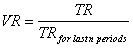Chart Pro online
User name (email):

 Recently used

 Forex

 Indices

# Volatility Ratio (VR)

Volatility Ratio (VR) is technical analysis indicator used to identify price ranges and breakouts.

Volatility ratio is calculated by formula:Calculation of VR is based on true range. For n parameter is usually used value of 14.Volatility Ratio (VR)

Interpretation

If value of volatility ratio is greater than 0.5 it is signal for breakout.

Example